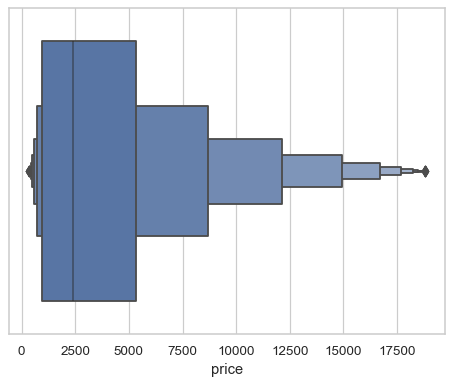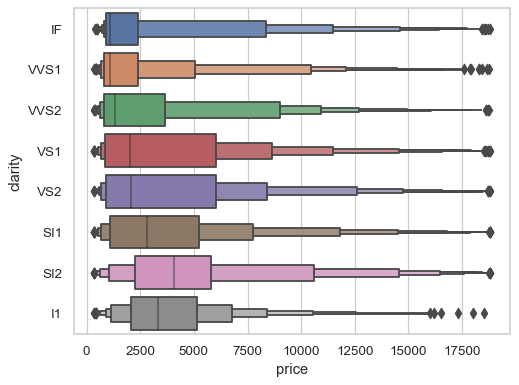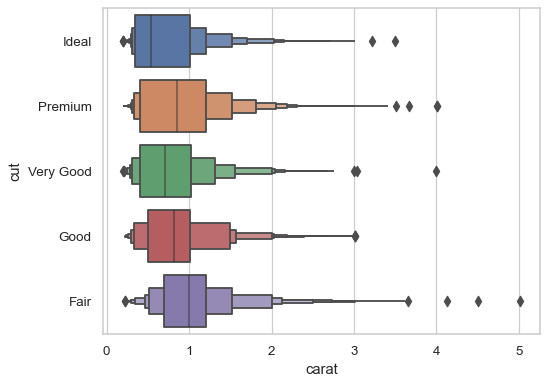# seaborn.boxenplot#

seaborn.boxenplot(data=None, *, x=None, y=None, hue=None, order=None, hue_order=None, orient=None, color=None, palette=None, saturation=0.75, width=0.8, dodge=True, k_depth='tukey', linewidth=None, scale='exponential', outlier_prop=0.007, trust_alpha=0.05, showfliers=True, ax=None, box_kws=None, flier_kws=None, line_kws=None)#

Draw an enhanced box plot for larger datasets.

This style of plot was originally named a “letter value” plot because it shows a large number of quantiles that are defined as “letter values”. It is similar to a box plot in plotting a nonparametric representation of a distribution in which all features correspond to actual observations. By plotting more quantiles, it provides more information about the shape of the distribution, particularly in the tails. For a more extensive explanation, you can read the paper that introduced the plot: https://vita.had.co.nz/papers/letter-value-plot.html

Note

This function always treats one of the variables as categorical and draws data at ordinal positions (0, 1, … n) on the relevant axis, even when the data has a numeric or date type.

Parameters:
dataDataFrame, array, or list of arrays, optional

Dataset for plotting. If `x` and `y` are absent, this is interpreted as wide-form. Otherwise it is expected to be long-form.

x, y, huenames of variables in `data` or vector data, optional

Inputs for plotting long-form data. See examples for interpretation.

order, hue_orderlists of strings, optional

Order to plot the categorical levels in; otherwise the levels are inferred from the data objects.

orient“v” | “h”, optional

Orientation of the plot (vertical or horizontal). This is usually inferred based on the type of the input variables, but it can be used to resolve ambiguity when both `x` and `y` are numeric or when plotting wide-form data.

colormatplotlib color, optional

Single color for the elements in the plot.

palettepalette name, list, or dict

Colors to use for the different levels of the `hue` variable. Should be something that can be interpreted by `color_palette()`, or a dictionary mapping hue levels to matplotlib colors.

saturationfloat, optional

Proportion of the original saturation to draw colors at. Large patches often look better with slightly desaturated colors, but set this to `1` if you want the plot colors to perfectly match the input color.

widthfloat, optional

Width of a full element when not using hue nesting, or width of all the elements for one level of the major grouping variable.

dodgebool, optional

When hue nesting is used, whether elements should be shifted along the categorical axis.

k_depth{“tukey”, “proportion”, “trustworthy”, “full”} or scalar

The number of boxes, and by extension number of percentiles, to draw. All methods are detailed in Wickham’s paper. Each makes different assumptions about the number of outliers and leverages different statistical properties. If “proportion”, draw no more than `outlier_prop` extreme observations. If “full”, draw `log(n)+1` boxes.

linewidthfloat, optional

Width of the gray lines that frame the plot elements.

scale{“exponential”, “linear”, “area”}, optional

Method to use for the width of the letter value boxes. All give similar results visually. “linear” reduces the width by a constant linear factor, “exponential” uses the proportion of data not covered, “area” is proportional to the percentage of data covered.

outlier_propfloat, optional

Proportion of data believed to be outliers. Must be in the range (0, 1]. Used to determine the number of boxes to plot when `k_depth="proportion"`.

trust_alphafloat, optional

Confidence level for a box to be plotted. Used to determine the number of boxes to plot when `k_depth="trustworthy"`. Must be in the range (0, 1).

showfliersbool, optional

If False, suppress the plotting of outliers.

axmatplotlib Axes, optional

Axes object to draw the plot onto, otherwise uses the current Axes.

box_kws: dict, optional

Keyword arguments for the box artists; passed to `matplotlib.patches.Rectangle`.

line_kws: dict, optional

Keyword arguments for the line denoting the median; passed to `matplotlib.axes.Axes.plot()`.

flier_kws: dict, optional

Keyword arguments for the scatter denoting the outlier observations; passed to `matplotlib.axes.Axes.scatter()`.

Returns:
axmatplotlib Axes

Returns the Axes object with the plot drawn onto it.

`violinplot`

A combination of boxplot and kernel density estimation.

`boxplot`

A traditional box-and-whisker plot with a similar API.

`catplot`

Combine a categorical plot with a `FacetGrid`.

Examples

```df = sns.load_dataset("diamonds")
```

Draw a single horizontal plot, assigning the data directly to the coordinate variable:

```sns.boxenplot(x=df["price"])
```Group by a categorical variable, referencing columns in a datafame

```sns.boxenplot(data=df, x="price", y="clarity")
```Use a different scaling rule to control the width of each box:

```sns.boxenplot(data=df, x="carat", y="cut", scale="linear")
```Use a different method to determine the number of boxes:

```sns.boxenplot(data=df, x="carat", y="cut", k_depth="trustworthy")
```